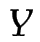## 9.4.3 Subscripts and Superscripts

Subscripts are obtained either by the command

\sb{<maths>}

or by the special character:

_{<maths>}

Superscripts are obtained either by the command

\sp{<maths>}

or by the special character:

^{<maths>}

Examples:

1. This example uses \sb and \sp:

2. This example uses _ and ^

$y = x_{1}^{2} + x_{2}^{2}$

3. Recall from earlier that mandatory arguments only consisting of one character don't need to be grouped, so the above code can also be written as:

$y = x_1^2 + x_2^2$

This is simpler than the first two examples. However it's a good idea to be in the habit of always using braces in case you forgot them when they're needed.

All three of the above examples produce the same output:Notice how the subscript gets tucked under the slope of thein:

$Y_{1}^{2}$Compare with

$Y{}_{1}^{2}$Example (Nested)

Subscripts and superscripts can also be nested (note that it is now necessary to group the argument to the superscript command):

$f(x) = e^{x_1}$

which producesThis example isn't quite right as e isn't actually a variable and shouldn't be typeset in italic. The correct way to do this is:

$f(x) = \mathrm{e}^{x_1}$

which results in:If you are going to use e a lot, it will be simpler to define a new command to do this. The definition should go in the preamble:

\newcommand{\e}{\mathrm{e}}

Then in the document:

$f(x_1, x_2) = \e^{x_1^2} + \e^{x_2^2}$Take care when nesting subscripts or superscripts. The following

x_1_2
will give a ! Double subscript error.

This book is also available as A4 PDF or 12.8cm x 9.6cm PDF or paperback (ISBN 978-1-909440-00-5).

© 2012 Dickimaw Books. "Dickimaw", "Dickimaw Books" and the Dickimaw parrot logo are trademarks. The Dickimaw parrot was painted by Magdalene Pritchett.